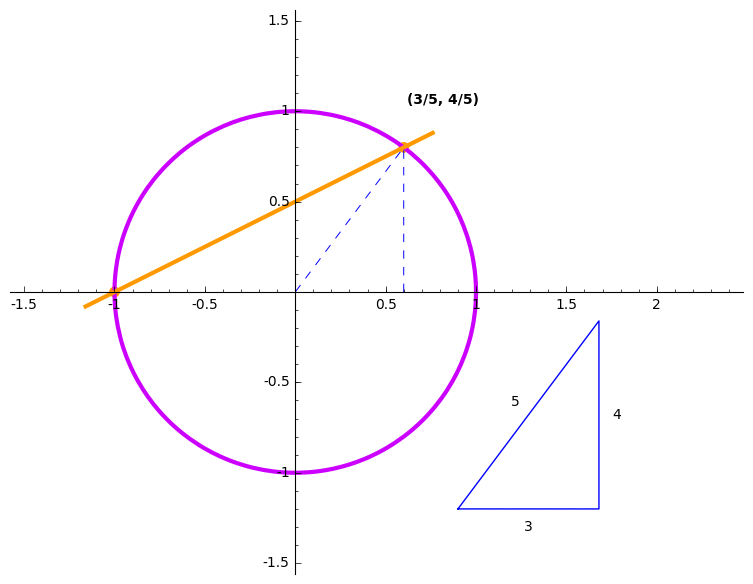# A formula for Pythagorean triples

A Pythagorean triple is a triple of whole numbers which fit to make the sides of a right triangle. For example, (3,4,5) is a Pythagorean triple, but (5,6,7) is not. That's because (3,4,5) satisfies the Pythagorean Theorem, but (5,6,7) does not:

$5^2 + 6^2 = 25 + 36 = 61$

but

$7^2 = 49 :($

## How can you come up with Pythagorean triples?

It's easy: pick a rational number t, like 1/2, or 5/7, whatever. Then make a point (x,y) like this:

$x = (1-t^2) / (1+t^2)$ $y = 2t / (1+t^2).$

One thing is obvious: If t is rational, then x and y are too. A second thing is not so obvious: (x,y) lies on the unit circle!! That's right:

$x^2 + y^2 = 1$

From this, you easily get a Pythagorean triple: Give x and y a common denominator, and then multiply both sides of the previous equation by the square of that denominator. You have a sum of two squares on the left, and another square on the right! The gif shows some examples of this.One way to check that this works is to take the formulas for x and y in terms of t, square them, and add them. After some algebraic simplification, you will see that the sum is 1. But this doesn't at all answer the question of where these formulas come from.

## Where do these formulas come from?!

This was completely opaque to me, but I read about a very clever idea:

Choose a rational number t. Draw a line which has slope t and passes through (-1,0). This line crosses the unit circle in some other point, q.

One thing is obvious about the other point q: It lies on the unit circle. A second thing is not so obvious: q has rational coordinates!! How do you know? Here's an explanation:

1. You know that q lies on the line y = t(x-1). This gives a formula for the y-coordinate of q.

2. Substitute this equation for the y-coordinate into the equation for points on the circle (remember that q definitely lies on the circle, so its coordinates satisfy this equation).

3. Treating t as a constant (some number), and x as a variable, you now have a quadratic equation in the variable x. Use the quadratic formula to solve it!! (Or just factor, using the fact that you already know one of the roots. If you don't already know one of the roots, don't worry, just use the quadratic formula and, when you're done, notice that you could have guessed one of those roots.)

4. Notice something special about the quadratic equation: When it gives two solutions, they are either both rational or both irrational. That's because the only possibly irrational part (assuming your coefficients were rational to begin with) is the part under the square root. Either that's a square and you get rational answers, or it's not, and you get two irrational answers.

5. This means that if your line has rational slope, and you know one of the points where it crosses the circle has rational coordinates, then the other one does too! In our case, one of the intersection points is (-1,0): definitely rational coordinates!

This explains why the second point has rational coordinates, even if you don't actually complete step (3) and find a formula for those coordinates in terms of the slope t. But if you do, guess what? It's the formula at the top of the article! So this idea about points where lines cross the circle leads to a formula for the previously mysterious Pythagorean triples!!

I find this connection between geometry and algebra breathtakingly beautiful. It's one of the starting points of the mathematical discipline called algebraic geometry. By doing more clever geometry, you can find interesting solutions to all kinds of fascinating equations.

p.s. I made the picture using Sage Mathematical Software System; you can take a look and modify to make your own!

p.p.s. If you read the Wikipedia article on Pythagorean triples, you'll see that this formula is basically the same as what's known as Euclid's Formula. The line drawing method I've described is equivalent to the stereographic projection described there (but starting at (0,1) instead of (-1,0)). But I don't think the equivalence of the two approaches is explained there. The part about using the structure of the quadratic formula to know that the other coordinates are rational is completely missing. If you're interested in this topic, that would be a useful thing to add to Wikipedia :)

tags: fun-topics